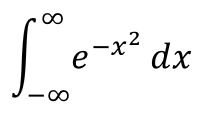## 单变量高斯积分- 阅读剩余部分 -

## 《随机行走人生路，量子计算是归途》《什么情况下冷水比热水升温快？》## 两带电球面之间的静电力- 阅读剩余部分 -

## 勒让德多项式递推公式的证明- 阅读剩余部分 -

## 勒让德多项式用勒让德多项式展开\begin{equation}
\varphi_0=\sum_{n=0}^{\infty}\left [a_n\left(\frac{r_p}{r}\right)^{n+1}P_n(\cos\theta) + A_n\left(\frac{R_p}{R}\right)^{n+1}P_n(\cos\Theta)\right ]
\label{elecpot}
\end{equation}

\begin{equation}
R^{-n-1}P_n(\cos\Theta)=(-1)^n\sum_{k=0}^{\infty}S^{-(k+n+1)}\frac{(k+n)!}{k!n!}r^k P_k(\cos\theta), r \lt S
\label{reexpansion1}
\end{equation}

\begin{equation}
r^{-n-1}P_n(\cos\theta)=\sum_{k=0}^{\infty}(-1)^kS^{-(k+n+1)}\frac{(k+n)!}{k!n!}R^kP_k(\cos\Theta), R \lt S
\label{reexpansion2}
\end{equation}

- 阅读剩余部分 -

## 从罗巨格公式推导勒让德多项式的递推关系- 阅读剩余部分 -